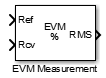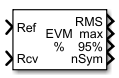# EVM Measurement

Measure error vector magnitude (EVM)

•Libraries:
Communications Toolbox / Utility Blocks

## Description

The EVM Measurement block measures the root mean squared (RMS) EVM, maximum EVM, and percentile EVM of a received signal. EVM is an indication of modulator or demodulator performance.

This icon shows the block with all ports enabled.## Ports

### Input

expand all

Reference signal, specified as an array of up to three dimensions. If you specify this input, the object measures the EVM of the `Rcv` input by using this input as a reference constellation.

The dimensions of this input must match those of the `Rcv` input. The object uses each element of this input as the reference symbol for the corresponding element of the `Rcv` input.

#### Dependencies

To enable this port, set the Reference signal parameter to `Input port`.

Data Types: `single` | `double` | `fixed point`
Complex Number Support: Yes

Received signal, specified as an array of up to three dimensions.

Data Types: `single` | `double` | `fixed point`
Complex Number Support: Yes

### Output

expand all

Percentage RMS EVM over the configured measurement interval, returned as a scalar.

Data Types: `double`

Maximum percentage EVM over the configured measurement interval, returned as a scalar.

#### Dependencies

To enable this port, set the Output maximum EVM parameter to `On`.

Data Types: `double`

Value below which X% of EVM measurements fall since the last reset, returned as a scalar in the range [0, 100]. Set the value of X in the X-percentile value (%) parameter.

#### Dependencies

To enable this port, set the Output X-percentile EVM parameter to `On`.

Data Types: `double`

Number of symbols used to measure the X-percentile EVM, returned as a positive integer.

#### Dependencies

To enable this port, set the Output X-percentile EVM and Output the number of symbols processed parameters to `On`.

Data Types: `double`

## Parameters

expand all

Normalization method used in EVM calculation, specified as `Average reference signal power`, `Average constellation power`, or `Peak constellation power`. For more information, see Algorithms.

Average constellation power in watts, specified as a positive scalar.

#### Dependencies

To enable this parameter, set the Normalize RMS error vector parameter to ```Average constellation power```.

Peak constellation power in watts, specified as a positive scalar.

#### Dependencies

To enable this parameter, set the Normalize RMS error vector parameter to ```Peak constellation power```.

Reference signal source. To provide an explicit reference signal against which to measure received signal, set this parameter to ```Input port```. To measure the EVM of the received signal against a reference constellation, set this parameter to ```Estimated from reference constellation```.

Reference constellation points, specified as a vector of complex or real values.

#### Dependencies

To enable this parameter, set the Reference signal parameter to ```Estimated from reference constellation```.

Measurement interval source for RMS and maximum EVM measurements, specified as one of these values.

• `Input length` — Measure the EVM using only the current samples.

• `Entire history` — Measure the EVM for all samples.

• `Custom` — Measure the EVM over an interval you specify and use a sliding window.

• `Custom with periodic reset` — Measure the EVM over an interval you specify and reset the block after measuring over each interval.

Custom measurement interval in samples, specified as a positive integer.

#### Dependencies

To enable this parameter, set the Measurement interval parameter to `Custom` or `Custom with periodic reset`.

Dimensions over which the block averages the EVM measurements, specified as an integer or row vector of integers in the range [1, 3]. For example, to average across the rows, set this parameter to `2`.

This block supports variable-size inputs of the dimensions across which the averaging takes place. However, the input size for the non-averaged dimensions must remain constant. For example, if the input has size `[1000 3 2]` and you set this parameter to ```[1 3]```, then the output size is `[1 3 1]` and the number of elements in the second dimension must remain fixed at `3`.

Option to add the `max` port to output maximum EVM measurements.

Option to add the `X%` port to output X-percentile EVM measurements. When you set this parameter to `On`, X-percentile EVM measurements persist until you reset the block. The block performs these measurements by using all of the input frames since the last reset. You can set the value of X in the X-percentile value (%) parameter.

Value below which X% of EVM measurements fall, specified as a scalar in the range [0, 100].

#### Dependencies

To enable this parameter, set the Output X-percentile EVM parameter to `On`.

Option to add the `nSym` port to output number of symbols used to measure the X-percentile EVM.

#### Dependencies

To enable this parameter, set the Output X-percentile EVM parameter to `On`.

Type of simulation to run, specified as ```Interpreted execution``` or `Code generation`.

• `Interpreted execution` — Simulate the model by using the MATLAB® interpreter. This option requires less startup time, but the speed of subsequent simulations is slower than with the ```Code generation``` option. In this mode, you can debug the source code of the block.

• `Code generation` — Simulate the model by using generated C code. The first time you run a simulation, Simulink® generates C code for the block. The model reuses the C code for subsequent simulations unless the model changes. This option requires additional startup time, but the speed of the subsequent simulations is faster than with the `Interpreted execution` option.

## Block Characteristics

 Data Types `double` | `fixed point` | `integer` | `single` Multidimensional Signals `yes` Variable-Size Signals `yes`

## Algorithms

The implementation supports three normalization methods. You can normalize measurements according to the average power of the reference signal, average constellation power, or peak constellation power. Different industry standards follow one of these normalization methods.

The algorithm calculates the RMS EVM value differently for each normalization method.

EVM Normalization MethodAlgorithm
Reference signal

`$EV{M}_{\text{RMS}}=\sqrt{\frac{\frac{1}{N}\sum _{k=1}^{N}\left({e}_{k}\right)}{\frac{1}{N}\sum _{k=1}^{N}\left({I}_{k}^{2}+{Q}_{k}^{2}\right)}}×100$`

Average power

`$EV{M}_{\text{RMS}}\left(\text{%}\right)=100\sqrt{\frac{\frac{1}{N}\sum _{k=1}^{N}\left({e}_{k}\right)}{{P}_{\text{avg}}}}$`

Peak power

`$EV{M}_{\text{RMS}}\left(\text{%}\right)=100\sqrt{\frac{\frac{1}{N}\sum _{k=1}^{N}\left({e}_{k}\right)}{{P}_{\text{max}}}}$`

In these equations:

• ek = ${e}_{k}={\left({I}_{k}-{\stackrel{˜}{I}}_{k}\right)}^{2}+{\left({Q}_{k}-{\stackrel{˜}{Q}}_{k}\right)}^{2}$

• Ik is the in-phase measurement of the kth symbol in the burst.

• Qk is the quadrature phase measurement of the kth symbol in the burst.

• N is the input vector length.

• Pavg is the average constellation power.

• Pmax is the peak constellation power.

• Ik and Qk represent ideal (reference) values. ${\stackrel{˜}{I}}_{k}$ and ${\stackrel{˜}{Q}}_{k}$ represent measured (received) symbols.

The maximum EVM is the maximum EVM value in a frame or $EV{M}_{\text{max}}=\underset{k\in \left[1,...,N\right]}{\text{max}}\left\{EV{M}_{k}\right\}\text{\hspace{0.17em}}\text{,}$ where k is the kth symbol in a burst of length N.

The definition for EVMk depends on which normalization method you select for computing measurements. The implementation supports these algorithms.

EVM Normalization MethodAlgorithm
Reference signal

`$EV{M}_{k}=\sqrt{\frac{{e}_{k}}{\frac{1}{N}\sum _{k=1}^{N}\left({I}_{k}^{2}+{Q}_{k}^{2}\right)}}×100$`

Average power

`$EV{M}_{k}=100\sqrt{\frac{{e}_{k}}{{P}_{\text{avg}}}}$`

Peak power

`$EV{M}_{k}=100\sqrt{\frac{{e}_{k}}{{P}_{\text{max}}}}$`

The implementation computes the X-percentile EVM by creating a histogram of the incoming EVMk values. This output provides the EVM value below which X% of the EVM values fall.

 IEEE Standard 802.16-2017. "Part 16: Air Interface for Broadband Wireless Access Systems." March 2018.

 3GPP TS 45.005 V8.1.0 (2008–05). "Radio Access Network: Radio transmission and reception".

 IEEE® Standard 802.11a™-1999. "Part 11: Wireless LAN Medium Access Control (MAC) and Physical Layer (PHY) specifications: High-speed Physical Layer in the 5 GHz Band." 1999.